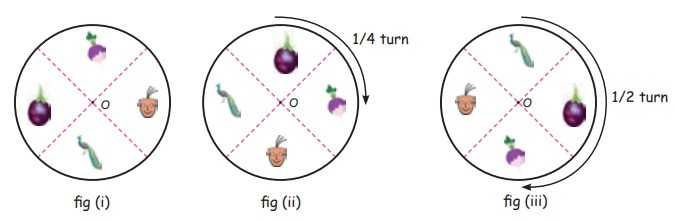Home | | Maths 5th Std | Able to explore rotations of familiar 2-D Shapes intuitively

# Able to explore rotations of familiar 2-D Shapes intuitively

Able to explore rotations of familiar 2-D Shapes intuitively.

Able to explore rotations of familiar 2-D Shapes intuitively.Draw two vertical lines in a white paper.

(By paper folding method)

Draw a circle with radius 6cm in a chart. Then Cut the circle from the chart. Fold it into four equal parts and spread again. Draw/paste four pictures on the circle as shown in the figure.Fix/put the round chart on the white paper by using a pin in center. By rotating the chart we observe that two vertical lines and the center point will lie at the same point. Look at the changes occurred, when the chart is rotated.

Turn the round chart as shown in the figure (ii). The picture in figure (i) is changed, as in figure (ii) This change is called as “rotation:. “O” is the “center”. When we compare the figures (i) and (ii) the pictures are changed as one-fourth turn. When we rotate the chart as shown in the figure (iii) the pictures are changed as half−turn.

To Observe

* When we rotate, the shapes are rotated.

* The images are rotated depending on the point.

* The point is called as “Centre of Rotation”

Think it: Is there any changes to the wings of the ventilater fan, after rotating one-fourth turn and half-turn.Example

Let's see the rotation of the square.Tags : Geometry | Term 1 Chapter 1 | 5th Maths , 5th Maths : Term 1 Unit 1 : Geometry
Study Material, Lecturing Notes, Assignment, Reference, Wiki description explanation, brief detail
5th Maths : Term 1 Unit 1 : Geometry : Able to explore rotations of familiar 2-D Shapes intuitively | Geometry | Term 1 Chapter 1 | 5th Maths